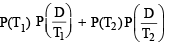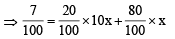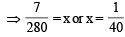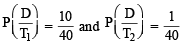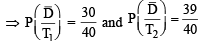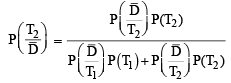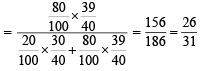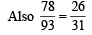Courses

# Test: Single Correct MCQs: Probability | JEE Advanced

## 27 Questions MCQ Test Mathematics For JEE | Test: Single Correct MCQs: Probability | JEE Advanced

Description
This mock test of Test: Single Correct MCQs: Probability | JEE Advanced for JEE helps you for every JEE entrance exam. This contains 27 Multiple Choice Questions for JEE Test: Single Correct MCQs: Probability | JEE Advanced (mcq) to study with solutions a complete question bank. The solved questions answers in this Test: Single Correct MCQs: Probability | JEE Advanced quiz give you a good mix of easy questions and tough questions. JEE students definitely take this Test: Single Correct MCQs: Probability | JEE Advanced exercise for a better result in the exam. You can find other Test: Single Correct MCQs: Probability | JEE Advanced extra questions, long questions & short questions for JEE on EduRev as well by searching above.
QUESTION: 1

### Two fair dice are tossed. Let x be the event that the first die shows an even number and y be the event that the second die shows an odd number. The two events x and y are :

Solution:

If two dice are tossed, the total number of sample space = 36

P(A)= Probability that the first die shows an even number = 18/36 = 1/2

P(B) = Probability that the second die shows an odd number = 18/36 = ½

P(A∩B) The probability of even on first die and odd on second die = 9/36 = ¼

P(A∩B) = ¼, and hence they are not mutually exclusive.

Also, P(A). P(B) = (½).(½) = ¼

Thus, P(A∩B) = P(A).P(B)

Hence, they are independent.

Hence, Option (4) is the correct answer.

QUESTION: 2

### Two events A an d B ha ve pr obabilities 0.25 an d 0.50 respectively. The probability that both A and B occur simultaneously is 0.14. Then the probability that neither A nor B occurs is (1980)

Solution:

P (A ∪ B) = P (A) + P (B) – P (A ∩ B) = 0.25 + 0.50 – 0.14 = 0.61
∴ P (A' ∩ B') = P ((A ∪ B)') = 1 – P (A ∪ B)
= 1– 0.61 = 0.39

QUESTION: 3

### The probability that an event A happens in one trial of an experiment is 0.4. Three independent trials of the experiment are performed. The probability that the event A happens at least once is (1980)

Solution:

p = 0.4, n = 3, P (X ≥ 1) = ? ⇒ q = 0.6
∴ P (X ≥ 1) = 1– P (X = 0)
= 1– 3C0(0.4)0 (0.6)3 = 1 – 0.216 = 0.784

QUESTION: 4

If A and B are two events such that P(A) > 0, and P(B) ≠ 1 ,then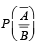is equal to (Here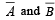are complements of A and B respectively).   (1982 - 2 Marks)

Solution: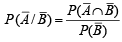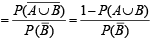QUESTION: 5

Fifteen coupons are numbered 1, 2 ..... 15,respectively. Seven coupons are selected at random one at a time with replacement. The probability  that the largest number appearing on a selected coupon is 9, is (1983 - 1 Mark)

Solution:

n = 7

Prob. of getting any no. out 1, 2, 3, … 9 is p = 9/15

∴ q = 6/5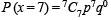[Binomial distribution]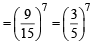QUESTION: 6

Three identical dice are rolled. The probability that the same number will appear on each of them is (1984 - 2 Marks)

Solution:

Favourable cases = 6; {(1, 1, 1), (2, 2, 2),…(6, 6, 6)}

Total cases  = 6 × 6 × 6 = 216
∴Req. prob. =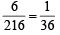QUESTION: 7

A box con t ain s 24 iden tical balls of wh ich 12 ar e wh ite and 12 are black. The balls are drawn at random from the box one at a time with replacement. The probability that a white ball is drawn for the 4th time on the 7th draw is (1984 - 2 Marks)

Solution:

Prob. of a getting a white ball in a single draw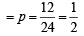Prob. of getting a white ball 4th time in the 7th draw = P (getting 3 W in 6 draws) × P (getting W ball at 7th draw)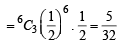QUESTION: 8

One hundred identical coins, each with probability, p, of showing up heads are tossed once. If 0 < p < 1 and the probabilitity of heads showing on 50 coins is equal to that of heads showing on 51 coins, then the value of p is (1988 - 2 Marks)

Solution:

Prob. of one coin showing head = p
∴ Prob of one coin showing tail = 1– p ATQ coin is tossed 100 times and prob. of 50 coins showing head = prob of 51 coins showing head.
Using binomial prob. distribution

P (X = r)= nCr prqn-r ,

we get,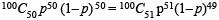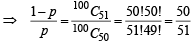⇒ 51 – 51 p = 50 p

⇒ 101 p = 51 ⇒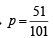QUESTION: 9

In dia plays two matches each with West In dies and Australia. In any match the probabilities of India getting, points 0, 1 and 2 are 0.45, 0.05 and 0.50 respectively.Assuming that the outcomes are independent, the probability of India getting at least 7 points is (1992 -  2 Marks)

Solution:

P (at least 7 pts) = P (7pts) + P (8 pts) [∵ At most 8 pts can be scored.]
Now 7 pts can be scored by scoring 2 pts in 3 matches and 1 pt. in one match, similarly 8 pts can be scored by scoring 2 pts in each of the 4 matches.
∴ Req. prob. = 4C1 × [P (2 pts)]3 P (1pt) + [P (2 pts)]4 = 4 (0.5)3 × 0.05 + (0.50)4  = 0.0250 + 0.0625 = 0.0875

QUESTION: 10

An unbiased die with faces marked 1, 2, 3, 4, 5 and 6 is rolled four times. Out of four face values obtained, the probability that the minimum face value is not less than 2 and the maximum face value is not greater than 5, is then: (1993 -  1 Mark)

Solution:

The min. face value is not less than 2 and max. face value is not greater than 5 if we get any of the numbers 2, 3, 4, 5, while total possible out comes are 1, 2 , 3, 4, 5 and 6.

∴ In one thrown of die, prob. of getting any no.
Out of 2, 3, 4 and 5 is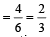If the die is rolled four times, then all these events being independent, the required prob.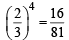QUESTION: 11

Let A , B , C be three mutually independent events.Consider the two statements S1 and S2 S1 : A and B ∪ C are independent S2 : A an d B ∩ C are independent Then,(1994)

Solution:

P [A ∩ (B ∪ C)] = P [(A ∩ B) ∪ (A ∩ C)] = P (A ∩ B) + P (A ∩ C) – P (A ∩ B ∩ C)
= P (A)  P (B) + P (A)  P (C) – P (A)  P (B) P(C)
= P (A) [P (B) + P (C) – P (B ∩ C)] = P (A) P (B ∪ C)

∴ S1 is true.
P (A ∩ (B ∩ C)) = P (A) P(B)  P (C) = P (A) P (B ∩ C)
∴ S2 is also true.

QUESTION: 12

The probability of India winning a test match against west Indies is 1/2. Assuming independence from match to match the probability that in a 5 match series India’s second win occurs at third test is (1995S)

Solution:

Given that P (India wins) = p = 1/2
∴ P (India loses) = p' = 1/2 Out of 5 matches india’s second win occurs at third test
⇒ India wins third test and simultaneously it has won one match from first two and lost the other.
∴ Required prob. = P (LWW) + P (WLW)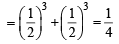QUESTION: 13

Three of the six vertices of a regular hexagon are chosen at random. The probability that the triangle with three vertices is equilateral, equals (1995S)

Solution:

Out of 6 vertices 3 can be chosen in 6C3 ways.
Δ will be equilateral if it is Δ ACE or ΔBDF (2 ways)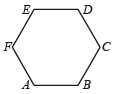∴ Required prob. =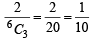QUESTION: 14

For the three events A, B, and C, P (exactly one of the events A or B occurs) = P (exactly one of the two events B or C occurs) = P(exactly one of the events C or A occurs) = p and P (all the three events occur simultaneously) = p2, where 0 < p < 1/2. Then the probability of at least one of the three events A, B and C occurring is (1996 - 2 Marks)

Solution:

We know that P (exactly one of A or B occurs)  = P (A) + P (B) – 2P (A ∩ B).
Therefore, P (A) + P (B) – 2P (A ∩ B) = p … (1)
Similarly,  P (B) + P (C) – 2P (B ∩ C) = p … (2)
and P (C) + P (A) – 2P (C ∩ A) = p …(3)
Adding (1), (2) and (3) we get 2 [P (A) + P (B) + P (C) – P (A ∩ B)
– P (B ∩ C) – P (C ∩ A)] = 3p ⇒ P (A) + P (B) + P (C) – P (A ∩ B)
– P (B ∩ C) – P (C ∩ A) = 3p/2 … (4)
We are also given that, P (A ∩ B ∩ C) = p2 … (5)
Now, P (at least one of A, B and C) = P (A) + P (B) + P (C) – P (A ∩ B) – P (B ∩ C)
– P (C ∩ A) + P (A ∩ B ∩ C)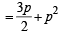[using (4) and (5)]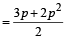QUESTION: 15

If the integers m and n are chosen at random from 1 to 100, then the probability that a number of the form  7m + 7n is divisible by 5 equals (1999 - 2 Marks)

Solution:

We know that, 71= 7, 72 = 49, 73 = 343, 74 = 2401, 75 = 16807
∴ 7k (where k ∈ Z), results in a number whose unit’ss digit is 7 or 9 or 3 or 1.
Now, 7m + 7n will be divisible by 5 if unit’s place digit of resulting number is 5 or 0 clearly it can never be 5.
But it can be 0 if we consider values of m and n such that the sum of unit’s place digits become 0. And this can be done by choosing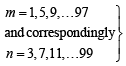(25 options each) [7 + 3 = 10]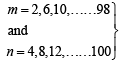(25 options each) [9 + 1= 10]

Case I : Thus m can be chosen in 25 ways and n can be chosen in 25 ways

Case II : m can be chosen in 25 ways and n can be chosen in 25 ways

∴ Total no. of selections of m, n so that 7m + 7n is divisible by 5 = (25 × 25 + 25 × 25 ) × 2

Note we can interchange values of m and n.
Also no. of total possible selections of m and n out of 100 = 100 × 100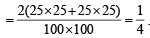QUESTION: 16

Two numbers are selected randomly from the set S = {1, 2, 3, 4, 5, 6} without replacement one by one. The probability that minimum of the two numbers is less than 4 is (2003S)

Solution:

The minimum of two numbers will be less than 4 if at least one of the numbers is less than 4.

∴ P (at least one no. is < 4), = 1– P (both the no’s are ≥ 4)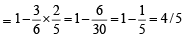QUESTION: 17

If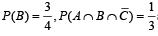and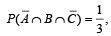then P (B∩C) is                     (2003S)

Solution:

Given that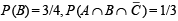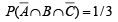From venn diagram, we see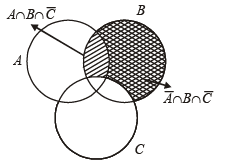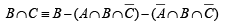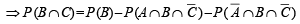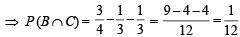QUESTION: 18

If three distinct numbers are ch osen randomly from the first 100 natural numbers, then the probability that all three of them are divisible by both 2 and 3 is (2004S)

Solution:

If a no. is to be divisible by both 2 and 3. It should be divisible by their L.C.M.
∴ L.C.M. of (2 and 3) = 6
∴ Numbers are  =  6, 12, 18 …  96.
Total numbers are = 16

∴ Probability =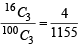QUESTION: 19

A six faced fair dice is thrown until 1 comes, then the probability that 1 comes in even no. of trials is (2005S)

Solution:

In single throw of a dice, probability of getting 1 is =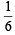and prob. of not getting 1 is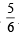Then getting 1 in even no. of chances = getting 1 in 2nd chance or in 4th chance or in 6th chance and so on

∴ Req. Prob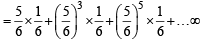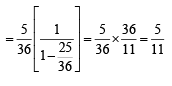QUESTION: 20

One Indian and four American men and their wives are to be seated randomly around a circular table. Then the conditional probability that the Indian man is seated adjacent to his wife given that each American man is seated adjacent to his wife is (2007 -3 marks)

Solution:

Let E1 ≡ The Indian man is seated adjacent to his wife.
E2 ≡ Each American man i s seated adja cen t to his wife.

Then P(E1 ∩ E2) =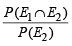Now E1 ∩E2≡ All men are seated adjacent to their wives.
∴ We can consider the 5 couples as single-single objects which can be arranged in a circle in 4! ways.
But for each couple, husband and wife can interchange their places in 2! ways.
∴ Number of ways when all men are seated adjacent to their wives = 4! × (2!)5
Also in all 10 persons can be seated in a circle in 9! ways.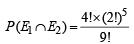Similarly if each American man is seated adjacent to his wife, considering each American couple as single object and Indian woman and man as seperate objects there are 6 different objects which can be arranged in a circle in 5! ways. Also for each American couple, husband and wife can interchange their places in 2 ! ways.
So the number of ways in which each American man is seated adjacent to his wife.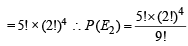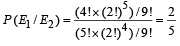QUESTION: 21

Let Ec denote the complement of an event E. Let E, F, G be pairwise independent events with P(G) > 0 and P(E∩F∩G) = 0. Then P(Ec∩ Fc| G) equals   (2007 -3 marks)

Solution:

P( Ec ∩ Fc /G) = P( E ∪ F )c /G) 1 - P(E∪ F /G)
= 1 - P(E / G) - P(F/ G) + P(E∩ F / G)
= 1 - P(E ) -P(F)+O
(∵ E, F, G are pairwise independent and
P(E ∩F ∩G)=0
⇒ P(E ).P(F )=0 as P(G) >0 ) = P(Ec )- P(F)

QUESTION: 22

An experiment has 10 equally likely outcomes. Let A and B be non-empty events of the experiment. If A consists of 4 outcomes, the number of outcomes that B must have so that A and B are independent, is     (2008)

Solution:

We have n (S) = 10, n(A) = 4
Let n(B) = x and  n(A ∩ B)  = y
Then for A and B to be independent events P(A ∩ B) = P(A) P(B)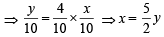⇒ y can be 2 or 4 so that x = 5 or 10
∴ n (B) = 5 or 10

QUESTION: 23

Let ω be a complex cube root of unity with ω ≠ 1. A fair die is thrown three times. If r1, r2 and r3 are the numbers obtained on the die, then the probability that ωr1 + ωr2 + ωr3 = 0 is (2010)

Solution:

If ω is a complex cube root of unity then, we know that ω3m 3n+13p +2 = 0 where m, n, p are integers.
∴ r1, r2, r3 should be of the form 3m, 3n + 1 and 3p + 2 taken in any or d er. As r1 ,r2 ,r3 are the numbers obtained on die, these can take any value from 1 to 6.
∴ m can take values 1 or 2, n can take values 0 or 1p can take values 0 or 1
∴ Number of ways of selecting r1, r2, r3
= 2C1 x 2C1 x 2C1 x 3!.
Also the total number of ways of getting r1, r2, r3 on die = 6 × 6 × 6

∴ Required probability=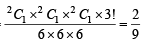QUESTION: 24

A signal which can be green or red with probability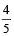and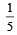respectively, is received by station A and then transmittedto station B. The probability of each station receiving the signal correctly is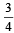.  If the signal received at station B is green, then the probability that the original signal was green is
(2010)

Solution:

Let G ≡ oreginal signal is green ⇒ P (G )= 45
E1 ≡ A receives the signal correctly P(E1) = 34
E2 ≡ B receives the signal correctly P(E2) = 34
E ≡ Signal received by B is green.
Then E can happen in the following ways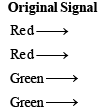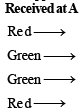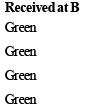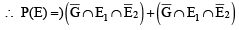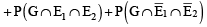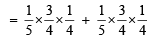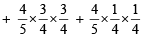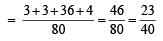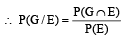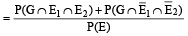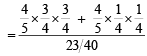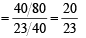QUESTION: 25

Four  fair  dice  D1, D2, D3 and  D4 ;  each  having  six  faces numbered  1,  2,  3,  4,  5  and  6  are  rolled simultaneously.
The probability that D4  shows a number appearing on one of D1, D2 and D3 is (2012)

Solution:

D4 can show an umber appearing on one of D1, D2 and D3 in the following cases.
Case I : D4 shows a number which is shown by only one of D1, D2 and D3.
D4 shows a number in 6C1 ways.
One out of D1, D2 and D3 can be selected in 3C1 ways.
The selected die shows the same number as on D4 in one way and rest two dice show the different number in 5 ways each.
∴ Number of ways to happen case I = 6C1 × 3C1 × 1 × 5 × 5 = 450
Case II : D4 shows a number which is shown by only two of D1, D2 and D3.
As discussed in case I, it can happen in the following number of ways = 6C1 × 3C2 × 1 × 1 × 5 = 90
Case III : D4 shows a number which is shown by all three dice D1, D2 and D3.
Number of ways it can be done = 6C1 × 3C3 × 1 × 1 × 1 = 6
∴ Total number of favourable ways = 450 + 90 + 6 = 546

Also total ways = 6 × 6 × 6 × 6

∴ Required Probability =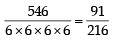QUESTION: 26

Three boys and two girls stand in a queue. The probability, that the number of boys ahead of every girl is at least one more than the number of girls ahead of her, is (JEE Adv. 2014)

Solution:

According to given condition , we can h ave the following cases
(I) G G B B B (II) B G G B B
(III) G B G B B (IV) B G B G B
(V) G B B G B
i.e., the two girls can occupy two of the first three places (case I, II, III) or second and fourth (case IV) or first and fourth (case V) places.
Thus favourable cases are = 3 × 2! × 3! + 2 × 2! × 3! = 60 Total ways in which 5 persons can be seated = 5! = 120
∴ Required probability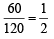QUESTION: 27

A computer producing factory has only two plants T1 and T2. Plant T1 produces 20% and plant T2 produces 80% of the total computers produced. 7% of computers produced in the factory turn out to be defective. It is known that P (computer turns out to be defective given that it is produced in plant T1) = 10P (computer turns out to be defective given that it is produced in plant T2), where P(E) denotes the probability of an event E. A computer produced in the factory is randomly selected and it does not turn out to be defective. Then the probability that it is produced in plant T2 is (JEE Adv. 2016)

Solution: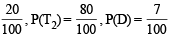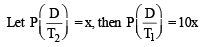Also P(D) =